# Domain and Range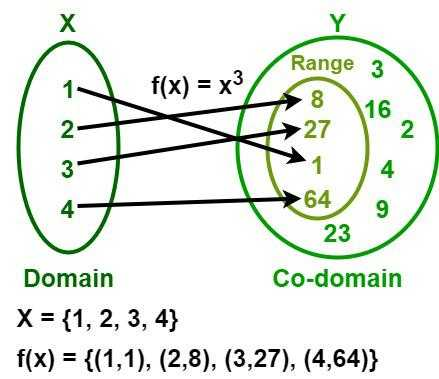Техника

## What is Domain and Range?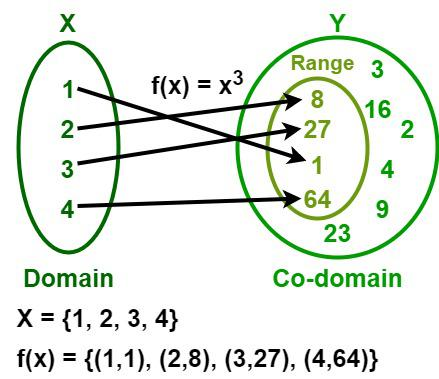For the given function f(x) = x3:

• f(x) = {(1,1), (2,8), (3,27), (4,64)}
• Domain = {1, 2, 3, 4}
• Co-domain = {1, 2, 3, 4, 8, 9, 16, 23, 27, 64}
• Range = {1, 8, 27, 64}

## Domain of a Function

The domain of a function is defined as the set of all possible values for which the function can be defined. Let us go through the domains of different functions.

• The domain of any polynomial function such as a linear function, quadratic function, cubic function, etc. is a set of all real numbers (R).
• The domain of a logarithmic function f(x) = log x is x > 0 or (0, ∞).
• The domain of a square root function f(x) = √x is the set of non-negative real numbers which is represented as [0, ∞).
• The domain of an exponential function is the set of all real numbers (R).
• A rational function is defined only for non-zero values of its denominator. So, to determine the domain of a rational function y = f(x), set the denominator ≠ 0.

## Rules of Finding Domain of a Function

Various rules for finding the domain of the function.

• Domain of the Polynomial functions (linear, quadratic, cubic, etc) function is R (all real numbers).
• Domain of the square root function √x is x ≥ 0.
• Domain of the exponential function is R.
• Domain of the logarithmic function is x > 0.
• We know that, the domain of a rational function y = f(x), denominator ≠ 0.

### How to Find the Domain of a Function?

Step 1: First, check whether the given function can include all real numbers.

Step 2: Then check whether the given function has a non-zero value in the denominator of the fraction and a non-negative real number under the denominator of the fraction.

Step 3: In some cases, the domain of a function is subjected to certain restrictions, i.e., these restrictions are the values where the given function cannot be defined. For example, the domain of a function f(x) = 2x + 1 is the set of all real numbers (R), but the domain of the function f(x) = 1/ (2x + 1) is the set of all real numbers except -1/2.

Step 4: Sometimes, the interval at which the function is defined is mentioned along with the function. For example, f (x) = 2x2 + 3, -5 < x < 5. Here, the input values of x are between -5 and 5. As a result, the domain of f(x) is (-5, 5).

After taking all the steps discussed above the set of numbers left with us is considered the domain of a function.

## Range of a Function

### Rules of Finding Range of a Function

Rules for finding the range of a function are,

• For linear function the range is R.
• For quadratic function y = a(x – h)2 + k the range is:
• y ≥ k, if a > 0
• y ≤ k, if a < 0
• For the square root function, the range is y ≥ 0.
• For the exponential function, the range is y > 0.
• For the logarithmic function, the range is R.

### How to Find the Range of a Function?

The range or image of a function is a subset of a co-domain and is the set of images of the elements in the domain.

Let us consider a function y = f(x).

Step 1: Write the given function in its general representation form, i.e., y = f(x).

Step 2: Solve it for x and write the obtained function in the form of x = g(y).

Step 3: Now, the domain of the function x = g(y) will be the range of the function y = f(x).

Thus, the range of a function is calculated.

Example: Find the range of the function f(x) = 1/ (4x − 3).

f(x) = 1/ (4x − 3).

Let the function be f(x) = y = 1/ (4x − 3).

y(4x − 3) = 1

4xy – 3y = 1

4xy = 1 + 3y

x = 4y / (1 + 3y)

Here, we observe that x is defined for all the values except of y for y = −1/3 as on y = -1/3, we get an undefined value of x.

So, the range of f(x) = 1/ (4x − 3) is (−∞, −1/3) U (1/3, ∞)

## Calculating Domain And Range

Domain = All the input values  = X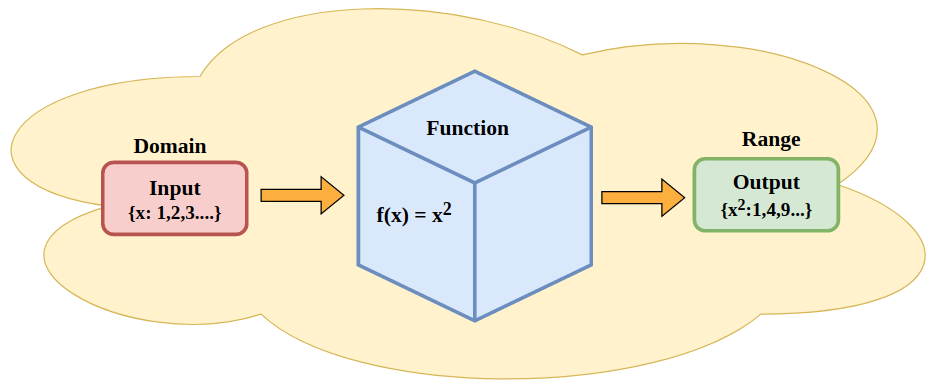Now domain and range of various functions are discussed below,

### Domain and Range of Exponential Functions

The exponential function is defined as,

The domain of the exponential function is all the real numbers and as the exponential function always gives the positive output, the range is the set of all the positive real numbers.

• Domain = R
• Range = R+

### Domain and Range of Trigonometric Functions

For trigonometric functions, the domain is a set of all real numbers (except some values in some functions) and the range of the trigonometric functions varies with different trigonometric functions, such that

• Range of Sine Function = [-1, 1]
• Range of Cosine Function = [-1, 1]
• Range of Cosecant Function = [-1, 1]
• Range of Secant Function = [-1, 1]

The range for Tangent and Cotangent functions is different,

• Range of Tangent Function = [-∞, ∞]
• Range of Cotangent Function = [-∞, ∞]

This can be summarized in the table below:

### Domain and Range of an Absolute Value Function

Absolute functions are the functions that are defined for all real numbers but their output is only positive real numbers, an absolute function only gives a positive output.

An absolute function is defined as,

• Domain = R
• Range = R+

### Domain and Range of a Square Root Function

For a square root function, the domain and range are calculated as,

Suppose the square root function is, f(x) = √(ax+b)

We know that the square root of a negative number is not defined, so the domain of the square root function is,

• Domainn = x ≥ -b/a = [-b/a,∞)

Now for the range of the square root function, we know that an absolute square root only gives positive values so the range is all positive real numbers.

• Range = R+

## Solved Example on Domain and Range

Example 1: Find the domain of a function f(x) = (2x + 1)/ (x2 − 4x + 3).

f(x) = (2x + 1)/ (x2 − 4x + 3)

f(x) = (2x + 1)/ (x − 1)(x − 3)

Observing the function we can say that the function f(x) is defined for all the values of x except for the values where, the denominator of the function is zero.

So f(x) is not defined when,

(x − 1)(x − 3) = 0

This can be acheived if of the bracket is zero, i.e.

x − 1 = 0 => x = 1 is where the function f(X) is undefined.

x − 3 = 0 => x = 3 is where the function f(X) is undefined.

Example 2: Find the domain and range of a function f(x) = x2 + 1.

f(x) = x2 + 1

This is a polynomial function and we know that a polynomial function is defined for all the values of x.

Thus, f(x) is defined for all x.

Domain of f(x) = R = (-∞, ∞)

Let f(x) = y = x2 + 1

y = x2 + 1

x2 = y − 1

x = √(y − 1)

The square root of the function is defined for all the vaues except for the negative values.

So, (y − 1) ≥ 0

Example 3: Find the domain and range of a function f(x) = (x + 2)/ (x – 3).

f(x) = (x + 2)/ (x – 3)

Observing the function we can say that the function f(x) is defined for all the values of x except for the values where, the denominator of the function is zero.

Thus the function is defined for all the values of x but not where x-3 = 0

x – 3 = 0

Let y = f(x)

y = (x + 2)/ (x – 3)

y(x – 3) = (x + 2)

xy – 3y = x + 2

xy – x = 3y + 2

x (y – 1) = 3y + 2

x = (3y + 2)/ (y – 1)

Observing the above equation we can say that x is defined for all the values except for the values where the denominator of the functiuon is zero, i.e.

y – 1 = 0

Example 4: Find the domain and range of a function f(x) = 3ex/7.

f(x) = 3ex/7

It is an exponential function which is defined for all the values of x.

So, Domain of f(x) is R

Let f(x) = y

y = 3ex/7

ex = 7y/3

x = loge(7y/3)

We know that logarithmic functions are defined only for the positive values of x.

So, x is defined only when y > 0

Thus, range of f(x) is (0, ∞)

## FAQs on Domain and Range

### Q1: Define a Function.

In mathematics, a function is defined as the relation between a set of inputs and their outputs, where the input can have only one output.

### Q3: Define the Domain and Give an Example.

The domain of a function is defined as the set of all possible values for which the function can be defined. The domain of any polynomial function such as a linear function, quadratic function, cubic function, etc. is a set of all real numbers (R).

### Q4: Define the Co-Domain and Range of a Function.

A co-domain of a function is the set of possible outcomes, whereas a range or image of a function is a subset of a co-domain and is the set of images of the elements in the domain.

• Дополнительно:  Magisk 26.1

Given an array A of N integers and number of queries Q. You have to answer two types of queries.

• Update [l, r] – for every i in range from l to r update Ai with sqrt(Ai), where sqrt(Ai) represents the square root of Ai in integral form.
• Query [l, r] – calculate the sum of all numbers ranging between l and r in array A.

Below is the implementation of the above approach:

## CPP

using namespace std;

const int MAX = 100;

    int type, l, r;

void update(int x, int val, int n)

int sum(int x)

    int s = 0;

    int q = 4;

    answerQueries(arr, que, n, q);

## Java

static int MAX = 100;

    public queries(int t_val, int l_val, int r_val)

        type = t_val;

        l = l_val;

        r = r_val;

    int s = 0;

    HashSet<Integer> s = new HashSet<Integer>();

                Stream<Integer> st = s.stream().filter(x -> x >= val);

                int it = st.findFirst().orElse(0);

    int q = 4;

    int n = arr.length;

        answerQueries(arr, que, n, q);

## Python3

from typing import List

from math import sqrt, floor

MAX = 100

    def __init__(self, type: int = 0, l: int = 0, r: int = 0) -> None:

        self.type = type

        self.l = l

        self.r = r

def update(x: int, val: int, n: int) -> None:

    while a <= n:

        a += a & -a

def sum(x: int) -> int:

        a -= a & -a

    s = set()

    for i in range(1, n):

    for i in range(q):

                ss = list(sorted(s))

if __name__ == "__main__":

    n = len(arr)

    answerQueries(arr, que, n, q)

## C#

    const int MAX = 100;

    static void update(int x, int val, int n)

    static int sum(int x)

        int s = 0;

                              int n, int q)

        var s = new HashSet<int>();

        int q = 4;

        int n = arr.Length;

        answerQueries(arr, que, n, q);

## Javascript

let MAX = 100;

let BIT = new Array(MAX + 1).fill(0);

function lower_bound(arr, ele)

    for (var i = 0; i < arr.length; i++)

    return arr.length - 1;

    constructor(type, l, r)

        this.type = type;

        this.l = l;

        this.r = r;

function update(BIT, x, val, n)

    var a = x;

    while (a <= n)

        a += (a & -a);

    var s = 0;

    var a = x;

    while (a > 0)

        a -= (a & -a);

function answerQueries(arr, que, n, q)

    let s = new Set();

    for (var i = 1; i < n; i++)

    for (var i = 0; i < q; i++)

                var ss = Array.from(s);

let q = 4;

let n = arr.length;

answerQueries(arr, que, n, q);

• Time Complexity: O(logN) per query
• Auxiliary Space: O(N)

Input:  arr[]   = {7, 2, 3, 0, 5, 10, 3, 12, 18};
query[] = [0, 4], [4, 7], [7, 8]

Output: Minimum of [0, 4] is 0
Minimum of [4, 7] is 3
Minimum of [7, 8] is 12

A simple solution is to run a loop from L to R and find the minimum element in the given range. This solution takes O(n) time to query in the worst case.
Another approach is to use Segment tree. With segment tree, preprocessing time is O(n) and time to for range minimum query is O(Logn). The extra space required is O(n) to store the segment tree. Segment tree allows updates also in O(Log n) time.

### Can we do better if we know that the array is static?

How to optimize query time when there are no update operations and there are many range minimum queries?
Below are different methods.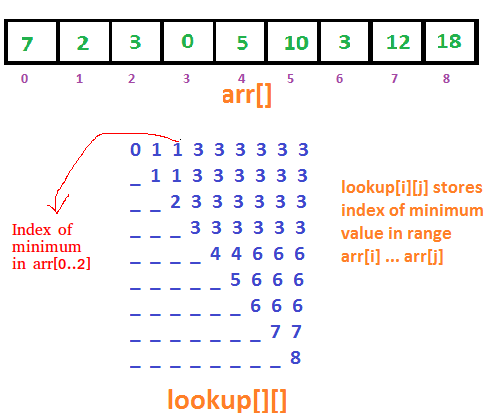## C++

using namespace std;

#define MAX 500

    int L, R;

    for (int i = 0; i < n; i++)

        for (int j = i + 1; j < n; j++)

    for (int i = 0; i < m; i++)

    RMQ(a, n, q, m);

## Java

    static int MAX = 500;

        int L, R;

        public Query(int L, int R)

            this.L = L;

            this.R = R;

        for (int i = 0; i < n; i++)

            for (int j = i + 1; j < n; j++)

        int n = a.length;

                      new Query(7, 8) };

        int m = q.length;

        RMQ(a, n, q, m);

## Python3

MAX = 500

    def __init__(self, L, R):

        self.L = L

        self.R = R

def preprocess(arr, n):

    for i in range(n):

    for i in range(n):

        for j in range(i + 1, n):

def RMQ(arr, n, q, m):

    for i in range(m):

if __name__ == "__main__":

    n = len(a)

    m = len(q)

    RMQ(a, n, q, m);

    static int MAX = 500;

        public int L, R;

        public Query(int L, int R)

            this.L = L;

            this.R = R;

        for (int i = 0; i < n; i++)

        for (int i = 0; i < n; i++)

            for (int j = i + 1; j < n; j++)

        int n = a.Length;

                      new Query(7, 8) };

        int m = q.Length;

        RMQ(a, n, q, m);

## Javascript

let MAX = 500;

let lookup = new Array(MAX);

for(let i = 0; i < MAX; i++)

    for(let j = 0; j < MAX; j++)

        this.L = L;

            this.R = R;

function preprocess(arr, n)

        for (let i = 0; i < n; i++)

            for (let j = i + 1; j < n; j++)

        for (let i = 0; i < m; i++)

let n = a.length;

let m = q.length;

RMQ(a, n, q, m);

Minimum of [0, 4] is 0
Minimum of [4, 7] is 3
Minimum of [7, 8] is 12

This approach supports queries in O(1), but preprocessing takes O(n2) time. Also, this approach needs O(n2) extra space which may become huge for large input arrays.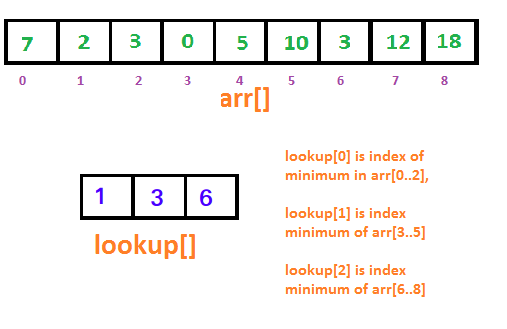// If arr[lookup] <=  arr[lookup],
// then lookup = lookup
If arr[lookup[i][j-1]] <= arr[lookup[i+2j-1][j-1]]
lookup[i][j] = lookup[i][j-1]

// If arr[lookup] >  arr[lookup],
// then lookup = lookup
Else
lookup[i][j] = lookup[i+2j-1][j-1]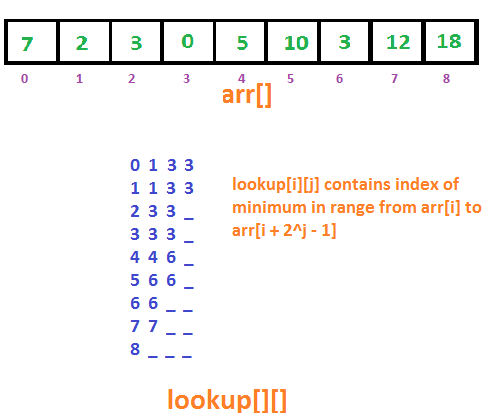// For (2,10), j = floor(Log2(10-2+1)) = 3
j = floor(Log(R-L+1))

// If arr[lookup] <=  arr[lookup],
// then RMQ(2,10) = lookup
If arr[lookup[L][j]] <= arr[lookup[R-(int)pow(2,j)+1][j]]
RMQ(L, R) = lookup[L][j]

// If arr[lookup] >  arr[lookup],
// then RMQ(2,10) = lookup
Else
RMQ(L, R) = lookup[R-(int)pow(2,j)+1][j]

Since we do only one comparison, the time complexity of the query is O(1).

Below is the implementation of the above idea.

## C++

using namespace std;

#define MAX 500

    int L, R;

    for (int i = 0; i < n; i++)

    for (int j = 1; (1 << j) <= n; j++)

        for (int i = 0; (i + (1 << j) - 1) < n; i++)

    int j = (int)log2(R - L + 1);

    for (int i = 0; i < m; i++)

             << query(arr, L, R) << endl;

    RMQ(a, n, q, m);

## Java

    static int MAX = 500;

        int L, R;

        public Query(int L, int R)

            this.L = L;

            this.R = R;

        for (int i = 0; i < n; i++)

        for (int j = 1; (1 << j) <= n; j++)

            for (int i = 0;

                 (i + (1 << j) - 1) < n;

        int j = (int)Math.log(R - L + 1);

        for (int i = 0; i < m; i++)

                               + L + ", " + R

                               + query(arr, L, R));

        int n = a.length;

                      new Query(7, 8) };

        int m = q.length;

        RMQ(a, n, q, m);

## Python3

from math import log2

MAX = 500

    def __init__(self, l, r):

        self.L = l

        self.R = r

def preprocess(arr: list, n: int):

    for i in range(n):

    while (1 << j) <= n:

        while i + (1 << j) - 1 < n:

            i += 1

        j += 1

def query(arr: list, L: int, R: int) -> int:

    j = int(log2(R - L + 1))

def RMQ(arr: list, n: int, q: list, m: int):

    for i in range(m):

              (L, R, query(arr, L, R)))

if __name__ == "__main__":

    n = len(a)

    m = len(q)

    RMQ(a, n, q, m)

    static int MAX = 500;

        public int L, R;

        public Query(int L, int R)

            this.L = L;

            this.R = R;

        for (int i = 0; i < n; i++)

        for (int j = 1; (1 << j) <= n; j++)

            for (int i = 0;

                 (i + (1 << j) - 1) < n;

        int j = (int)Math.Log(R - L + 1);

        for (int i = 0; i < m; i++)

        int n = a.Length;

                      new Query(7, 8) };

        int m = q.Length;

        RMQ(a, n, q, m);

## Javascript

const MAX = 500;

let lookup = new Array(MAX).fill(0).map(() => new Array(MAX))

        this.L = L;

        this.R = R;

    for (let i = 0; i < n; i++)

        for (let i = 0;

            (i + (1 << j) - 1) < n;

    let j = Math.floor(Math.log(R - L + 1));

            + L + ", " + R

            + query(arr, L, R) + "<br>");

let n = a.length;

let m = q.length;

RMQ(a, n, q, m);

Minimum of [0, 4] is 0
Minimum of [4, 7] is 3
Minimum of [7, 8] is 12

For the domain, we start by restricting the square root to be non-negative. After factorising, it becomes $(x+\sqrt2)(x-\sqrt2)\le0$. This is my explanation.

Дополнительно:  Что будет если убрать root права

I tried to substitute 2 as the value of y but it is wrong. Can anyone care to explain how he derived the domain from the factorisation and range?asked Sep 2, 2016 at 11:47The correct factorization is

answered Sep 2, 2016 at 12:03Peter Melech

a bit of rearranging and out pops the domain limits.
$$2 \geq x^2$$

answered Sep 2, 2016 at 12:28Ben Crossley

answered Sep 2, 2016 at 12:32Please guide how to find range of this functionasked Apr 3, 2013 at 2:37HINT: Note that

answered Apr 3, 2013 at 2:43Brian M. ScottBrian M. Scott

You have the domain correct; how does $y$ behave within this domain? Make a table of values, or plot it.

$$y(1) = y(5) = 2$$

answered Apr 3, 2013 at 2:44Ron Gordon

## Definitions of Domain and Range

### Domain

The domain of a
function is the complete set of possible values
of the independent variable.

In plain English, this definition means:

The domain is the set of all possible
x-values which will make the function
«work», and will output real y-values.

When finding the domain, remember:

• The denominator (bottom) of a fraction cannot be
zero
• The number under a square root sign must be
positive
in this section

## Domain & Range Math Problem Solver

This tool combines the power of mathematical computation engine that excels at solving mathematical formulas with the power of GPT large language models to parse and generate natural language. This creates math problem solver thats more accurate than ChatGPT, more flexible than a calculator, and faster answers than a human tutor.

### Domain and Range Interactive

After finishing this lesson head over to our interactive calculator to help you find the Domain and Range of a Fuction.

### Example 1a

Here is the graph of y = sqrt(x+4):

The domain of this function is x ≥ −4, since x cannot be less than  −4. To see why, try out some numbers less than −4 (like  −5 or  −10) and some more than −4 (like  −2 or 8) in your calculator. The only ones that «work» and give us an answer are the ones greater than or equal to  −4. This will make the number under the square root positive.

1. The enclosed (colored-in) circle on the point (-4, 0). This indicates that the domain «starts» at this point.
2. We saw how to draw similar graphs in section 4, Graph of a Function. For a more advanced discussion, see also How to draw y^2 = x − 2.

## How to find the domain

In general, we determine the domain of each function by looking for those values of the independent variable (usually x) which we are allowed to use. (Usually we have to avoid 0 on the bottom of a fraction, or negative values under the square root sign).

### Range

The range of
a function is the complete set of all possible
resulting values of the dependent variable (y, usually), after we have substituted the domain.

In plain English, the definition means:

The range is the resulting
y-values we get after substituting all the possible x-values.

## How to find the range

• The range of a function is the spread of possible y-values (minimum y-value to maximum y-value)
• Substitute different x-values into the expression for y to
see what is happening. (Ask yourself: Is y always positive? Always negative? Or maybe not equal to certain values?)
• Make sure you look for minimum and maximum values of y.
• Draw a sketch! In math, it’s very true that a picture is worth a thousand words.

### Example 1b

Let’s return to the example above, y = sqrt(x + 4).

We notice the curve is either on or above the horizontal axis. No matter what value of x we try, we will always get a zero or positive value of y. We say the range in this case is y ≥ 0.

The curve goes on forever vertically, beyond what is shown on the graph, so the range is all non-negative values of y.

### Example 2

The graph of the curve y = sin x shows the range to be betweeen −1 and 1.

The domain of y = sin x is «all values of x«, since there are no restrictions on the values for x. (Put any number into the «sin» function in your calculator. Any number should work, and will give you a final answer between −1 and 1.)

From the calculator experiment, and from observing the curve, we can see the range is y betweeen and . We could write this as −1 ≤ y ≤ 1.

Where did this graph come from? We learn about sin and cos graphs later in Graphs of sin x and cos x

Note 1: Because we are assuming that only real numbers are to be used for the x-values, numbers that lead to division by zero or to imaginary numbers (which arise from finding the square root of a negative number) are not included. The Complex Numbers chapter explains more about imaginary numbers, but we do not include such numbers in this chapter.

Дополнительно:  Root для oneplus one на 4pda

Note 2: When doing square root examples, many people ask, «Don’t we get 2 answers, one positive and one negative when we find a square root?» A square root has at most one value, not two. See this discussion: Square Root 16 — how many answers?

Note 3: We are talking about the domain and range of functions, which have at most one y-value for each x-value, not relations (which can have more than one.).

## Finding domain and range without using a graph

It’s always a lot easier to work out the domain and range when reading it off the graph (but we must make sure we zoom in and out of the graph to make sure we see everything we need to see).
However, we don’t always have access to graphing software, and sketching a graph usually requires knowing about discontinuities and so on first anyway.

As meantioned earlier, the key things to check for are:

1. There are no negative values under a square root sign
2. There are no zero values in the denominator (bottom) of a fraction

### Example 3

Find the domain and range of the function f(x)=sqrt(x+2)/(x^2-9), without using a graph.

#### Solution

In the numerator (top) of this fraction, we have a square root. To make sure the values under the square root are non-negative, we can only choose x-values grater than or equal to -2.

The denominator (bottom) has x^2-9, which we recognise we can write as (x+3)(x-3). So our values for x cannot include -3 (from the first bracket) or 3 (from the second).

We don’t need to worry about the -3 anyway, because we dcided in the first step that x >= -2.

To work out the range, we consider top and bottom of the fraction separately.

Numerator: If x=-2, the top has value sqrt(2+2)=sqrt(0)=0. As x increases value from -2, the top will also increase (out to infinity in both cases).

Denominator: We break this up into four portions:

When x=-2, the bottom is (-2)^2-9=4-9=-5. We have f(-2) = 0/(-5) = 0.

Between x=-2 and x=3, (x^2-9) gets closer to 0, so f(x) will go to -oo as it gets near x=3.

For x>3, when x is just bigger than 3, the value of the bottom is just over 0, so f(x) will be a very large positive number.

For very large x, the top is large, but the bottom will be much larger, so overall, the function value will be very small.

Have a look at the graph (which we draw anyway to check we are on the right track):

## Summary

In general, we determine the domain by
looking for those values of the independent variable (usually x) which we are allowed to use. (We have to avoid 0 on the bottom of a fraction, or negative values under the square root sign).

The range is found by finding the resulting y-values after we have substituted in the possible x-values.

### Exercise 1

(a) f(x) =
x^2+ 2.

Domain: The function

f(x) = x2 + 2

is defined
for all real values of x (because there are no restrictions on the value of x).

Hence, the domain of f(x) is

«all real values of x«.

Range: Since x2 is never negative,
x2 + 2 is never less than 2

Hence, the range of f(x) is

«all real numbers f(x) ≥ 2».

We can see that x can take any value in the graph, but the resulting y = f(x) values are greater than or equal to 2.

Domain: All x

1. It is important to label the axes when sketching graphs. It helps with understanding what the graph represents.
2. We saw how to sketch such graphs in Graph of a Function.

Domain: The function

is not defined for t =
-2, as this value would result in division by zero. (There would be a 0 on the bottom of the fraction.)

Hence the domain of f(t) is

«all
real numbers except -2»

Range: No matter how large or small t becomes,
f(t) will never be equal to zero.

Multiply both sides by (t + 2) and we get

0 = 1

So the range of f(t) is

«all
real numbers except zero».

We can see in the graph that the function is not defined for t = -2 and that the function (the y-values) takes all values except 0.

Domain: All t ≠ -2

Range: All f(t) ≠ 0

is not defined for real
numbers greater than 3, which would result in imaginary values
for g(s).

Hence, the domain for g(s) is
«all real numbers, s ≤ 3″.

Also, by definition,

g(s)=sqrt(3-s) >= 0

Hence, the range of g(s) is «all
real numbers g(s) ≥ 0»

We can see in the graph that s takes no values greater than 3, and that the range is greater than or equal to 0.

Domain: All s <= 3

Range:
All g(s) >= 0

(d) f(x) =
x^2+ 4 for
x > 2

The function f(x) has a domain of
«all real numbers, x > 2» as defined in the question. (There are no resulting square roots of negative numbers or divisions by zero involved here.)

To find the range:

• When x = 2, f(2) = 8
• When x increases from 2, f(x) becomes
larger than 8 (Try substituting in some numbers to see why.)

Hence, the range is «all real numbers, f(x) > 8»

Here is the graph of the function, with an open circle at (2, 8) indicating that the domain does not include x = 2 and the range does not include f(2) = 8.

Domain: All x>2

Range:
All f(x) > 8

### Exercise 2

We fire a ball up in the air and find the
height h, in metres, as a function of time
t, in seconds, is given by

h = 20t − 4.9t2

Find the domain and range for the function
h(t).

Generally, negative values of time do not have any
meaning. Also, we need to assume the projectile hits the ground and then stops — it does not go underground.

So we need to calculate when it is going to hit the ground. This will be when h = 0. So we solve:

20t − 4.9t2 = 0

(20 − 4.9t)t = 0

This is true when

t = 0\ «s»,

t=20/4.9 = 4.082 text(s)

Hence, the domain of the function h is

«all real
values of t such that 0 ≤ t ≤ 4.082»

We can see from the function expression that it is a parabola with its vertex facing up. (This makes sense if you think about throwing a ball upwards. It goes up to a certain height and then falls back down.)

What is the maximum value of h? We use the formula for maximum (or minimum) of a quadratic function.

The value of t that gives the maximum is

t = -b/(2a) = -20/(2 xx (-4.9)) = 2.041 s

So the maximum value is

20(2.041) − 4.9(2.041)2 = 20.408 m

By observing the function of h, we see that as t increases, h first increases to a maximum
of 20.408 m, then h decreases again to zero, as expected.

Hence, the range of h is

«all real
numbers, 0 ≤ h ≤ 20.408»

Here is the graph of the function h:

## Functions defined by coordinates

Sometimes we don’t have continuous functions. What do we do in this case? Let’s look at an example.

### Exercise 3

Find the domain and range of the function defined by the coordinates:

Here is the graph of our discontinuous function.

Оцените статью